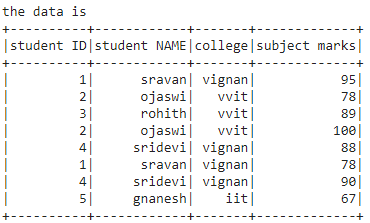Related Articles

# How to count unique ID after groupBy in PySpark Dataframe ?

• Last Updated : 17 Jun, 2021

In this article, we will discuss how to count unique ID after group by in PySpark Dataframe.

For this, we will use two different methods:

• Using distinct().count() method.
• Using SQL Query.

But at first, let’s Create Dataframe for demonstration:

## Python3

 `# importing module``import` `pyspark`` ` `# importing sparksession from pyspark.sql``# module``from` `pyspark.sql ``import` `SparkSession`` ` `# creating sparksession and giving an app name``spark ``=` `SparkSession.builder.appName(``'sparkdf'``).getOrCreate()`` ` `# list  of students  data``data ``=` `[[``"1"``, ``"sravan"``, ``"vignan"``, ``95``],``        ``[``"2"``, ``"ojaswi"``, ``"vvit"``, ``78``],``        ``[``"3"``, ``"rohith"``, ``"vvit"``, ``89``],``        ``[``"2"``, ``"ojaswi"``, ``"vvit"``, ``100``],``        ``[``"4"``, ``"sridevi"``, ``"vignan"``, ``88``],``        ``[``"1"``, ``"sravan"``, ``"vignan"``, ``78``],``        ``[``"4"``, ``"sridevi"``, ``"vignan"``, ``90``],``        ``[``"5"``, ``"gnanesh"``, ``"iit"``, ``67``]]`` ` `# specify column names``columns ``=` `[``'student ID'``, ``'student NAME'``,``           ``'college'``, ``'subject marks'``]`` ` `# creating a dataframe from the lists of data``dataframe ``=` `spark.createDataFrame(data, columns)`` ` `print``(``"the data is "``)``dataframe.show()`

Output:Method 1 : Using groupBy() and distinct().count() method

groupBy(): Used to group the data based on column name

Syntax: dataframe=dataframe.groupBy(‘column_name1’).sum(‘column name 2’)

distinct().count(): Used to count and display the distinct rows form the dataframe

Syntax: dataframe.distinct().count()

Example 1:

## Python3

 `# group by studentID by marks ``dataframe ``=` `dataframe.groupBy(``  ``'student ID'``).``sum``(``'subject marks'``)`` ` `# display count of unique ID``print``(``"Unique ID count after Group By : "``,``      ``dataframe.distinct().count())`` ` `print``(``"the data is "``)`` ` `# display  values  of unique ID``dataframe.distinct().show()`

Output:

```Unique ID count after Group By :  5
the data is
+----------+------------------+
|student ID|sum(subject marks)|
+----------+------------------+
|         3|                89|
|         5|                67|
|         1|               173|
|         4|               178|
|         2|               178|
+----------+------------------+```

Example 2: Count and display a unique ID of single columns:

## Python3

 `# group by studentID by marks ``dataframe ``=` `dataframe.groupBy(``  ``'student ID'``).``sum``(``'subject marks'``)`` ` `# display count of unique ID``print``(``"Unique ID count after Group By : "``,``      ``dataframe.distinct().count())`` ` ` ` `print``(``"the data is "``)`` ` `# display  values  of unique ID``dataframe.select(``'student ID'``).distinct().show()`

Output:

```Unique ID count after Group By :  5
the data is
+----------+
|student ID|
+----------+
|         3|
|         5|
|         1|
|         4|
|         2|
+----------+```

Method 2: Using SQL query

We can get a unique ID count by using spark.sql

Syntax:

spark.sql(“sql query”).show()

## Python3

 `# importing module``import` `pyspark`` ` `# importing sparksession from pyspark.sql ``# module``from` `pyspark.sql ``import` `SparkSession`` ` `# creating sparksession and giving an app name``spark ``=` `SparkSession.builder.appName(``'sparkdf'``).getOrCreate()`` ` `# list  of students  data``data ``=` `[[``"1"``, ``"sravan"``, ``"vignan"``, ``95``],``        ``[``"2"``, ``"ojaswi"``, ``"vvit"``, ``78``],``        ``[``"3"``, ``"rohith"``, ``"vvit"``, ``89``],``        ``[``"2"``, ``"ojaswi"``, ``"vvit"``, ``100``],``        ``[``"4"``, ``"sridevi"``, ``"vignan"``, ``88``],``        ``[``"1"``, ``"sravan"``, ``"vignan"``, ``78``],``        ``[``"4"``, ``"sridevi"``, ``"vignan"``, ``90``],``        ``[``"5"``, ``"gnanesh"``, ``"iit"``, ``67``]]`` ` `# specify column names``columns ``=` `[``'student ID'``, ``'student NAME'``,``           ``'college'``, ``'subject marks'``]`` ` `# creating a dataframe from the lists of data``dataframe ``=` `spark.createDataFrame(data, columns)`` ` `# group by studentID by marks``dataframe ``=` `dataframe.groupBy(``'student ID'``).``sum``(``'subject marks'``)`` ` `# create view for the ablve dataframe and``# view name is "DATA"``dataframe.createOrReplaceTempView(``"DATA"``)`` ` `# count unique data with sql query``spark.sql("SELECT DISTINCT(COUNT(``'student ID'``))  \``FROM DATA GROUP BY ``'subject marks'``").show()`

Output:

```+-----------------+
|count(student ID)|
+-----------------+
|                5|
+-----------------+```

Attention geek! Strengthen your foundations with the Python Programming Foundation Course and learn the basics.

To begin with, your interview preparations Enhance your Data Structures concepts with the Python DS Course. And to begin with your Machine Learning Journey, join the Machine Learning – Basic Level Course

My Personal Notes arrow_drop_up